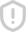### 非线性分析答案 评分=(…,y -1;0,y1,…,yn…)有: 0.土1.±2 ±( 再由定理(2)知:t(0(x),a(y)≤2≤e 证毕 2)证明类似1) 5证明双边符号动力系统(Σ,Z,a)是 Devaney意义下混沌的 证明 (证明分为三步 1)证明a的周期点在∑中稠密 2)r∈习,σ是对初值敏感依赖的 3)证明系统是拓扑传递的) 1)证明σ的周期点在∑中稠密 对vx0∈∑,记x0-( 任取>0,总存在N∈N+,使得3<.则取m=(…,b-m,…,b-1;bo,b, 其中b+k(2N+3) 0,±1士2…,±(N+1),k∈Z.x为2+3周期点,且 由上题1)中给出的定理知,d(x:x0)≤<E 即在∑的任意点的任意小领域中,都有周期点故周期点在∑中稠密 2)r∈,a是对初值敏感依赖的 任取xo∈∑xo=( 取0=2.对v6≥0,总存在N>0,使得2<6 取=(…,b 其中h2 0.+1.+ 1),但b+2≠aN+2,则由上题1)中给出的定理知,d(an.)≤<6. 作我们取k=N+2,则有d(aN+2(x0),N+2(W)2(+x+2=1>号=E0 由定义知σ是对初值敏感依赖的. 3)证明系统是拓扑传递的 设该符号空间有n个符号(n为有限正整数) 则将长度为1的n个符号的排列的所有可能情况顺次排成一列,该序列记为X1 将长度为2的n个符号的排列的所有可能情况顺次排成一列,该序列记为x2. 依次类推,我们可以得到Xk,由于n的有限性,故对k,Xk是有限长度的序列 我们取m=(…:X1,X2,……,H1,…).即从0位置依次正向排列Xk,由于Xk长 度有限,故这一步是可以执行的对于其他位置,我们可以任意取值 下证,x的轨道在∑中稠密 实际上,任取x0∈∑,记xo=(…,a-n,,a-1;a0,a1,…,an,…),则对v> 0,总存在N∈N+,使得,<E 而长度为2N+3的符号串(a(N-1,…,a-1:a0,a,…,aN+1),必然属于x2x+3 故存在k∈N+,使得a(x)-(…,b-n,…,b-1;bo,b1…,bn,…),其中b a,=0,±1,±2,…,±(N+1) 再由上题1)中给出的定理知,d(mk(x),x)≤2<E.即x的任意一个小领域内 都存在x的轨道中的点,故的轨道在∑中桐密 6对映射L1( ,证明(0,0)是渐进稳定的且它的吸引域为R2 证明 求映射在(0,0)处的10阵A=/20 0 容易看出A的特征值为,故谱半径为<1 所以(0,0)是渐进稳定的 0 L1( 2 故对 ∈B lim Ii(a)=lim/270 即(0,0)处的吸引域为R n→} 2→}。 0 0 7求 henon映射的不动点,并判断不动点的性态求出其中鞍点的稳定流形或者 不稳定流形. 1+ Henon块射:H( ∈P2,,b∈R (此题比较复杂,我只简单讨论了其中一个点的全部情况,等我研究一下再看怎 么弄,因为要求解稳定流形,这题的篇嘔就有些过长了.)解 令 1+ byy y +(1-b)x-1=0 当(1-b) 0时,尤不动点 当(1-b2+4a=0时,有唯一不动点为(24,b2 当(1-b)2+4a>0时,有两个不动点分别为(x1,m) (n29)-(21Y+,2=Y+ 先考虑(1-b)2+4a>0时不动点(x1,y1)的性态 2a b 映射的 jacobi矩阵为: 则在不动点(x1,)处的 jacobi矩阵的特征方程为:x2+2ax1A-b=0.若方程 有一个根的模为1分为以下两种情况 凊况1:有复根,则两根模必相等,等价于△=(2ax1)2+4b<0且b=-1 解得:-1<a<3b--1 情况2:无复根,则等价于1+2ax1-b=0或者1-2a1-b=0.解得:a (1-b) 或者a-.而(1-b)2+4a>0,故第一种解的情形不存在 上述情况的不动点直接判定性态比较困难,需要进一部分分析. 引入一个引理: 引理:实系数方程κ2-Wx-c=0的两根的模均小」等」1的充要条件为:b≤ 如果两根的模均小于1.则等价于满足引理的充要条件,且不满足某根的模为1的 情况. 由充要条件知:|2ax1≤1-b,6≤1并且有(1-b)2+4a>0 解得b≤1且 3(1-b) 再去掉某根的模为1的情况,得到:b<1且一D<a<4b. 我们再考虑两根的模都大于1的情况 若两根的模都大于等于1,则其根的倒数的模小于等于1大于0.此时的b必不为0 则我们考虑方程2-2b1入-若=0则此方程的根恰为入2+201-b=0的根 的倒数 则此方程的根的模小」等」1(注意此方程的根不会为0)等价」入2+2a1入 b=0的根的模都大于1. 再利用引理,有 <1 <1 解得:a (1-b且b≥ 或者α≤》且b<-1.其中第一种解舍去再大掉某根模为1的情况,并综 合(1-b)2+4a>0.有 3(1-b) 且b 其他情况下是一个根的模大 个根的模小」1的情况,此时是鞍点 8.1)求帐篷映射r的三周期点;2)分析帐篷映射T上的所有有理点是什么类型的 点 解 (这里我们更加一般的讨论n周期点的情形.(证明课上给的周期点定理 先证明::满足T(ax)=x当且仅当在符号空间里ax形式为 ,0,r:1,r 或=( 0. C1,C2 0…) 其中充分性是明显成立的(带入验证即可),下证必要性:记x=(x1,x2,x3,…,xm,…), 由于T"(r) T )2.当xn=0时 T(in,x+1,…)=(n+1,n+2,…)2 时 (xm,xn+1,…)2)=(xm+1,xn+2,…)2 则若c为n周期点,那么必有r(x)= 若xn=0,x为m周期点则必有x2+n=2,Y∈N+,此时的x的形式为x 0. 0,…) 若xn=1,x为n周期点则必有x-n=x;,Y∈N+.此时的x的形式为 rn-1,1,1,2,…·,n-1,0,1,2,…,n-1,1,1,72,……,mn-1,0 满足r3(x)=x形式应该是:(x1,x2,0,x1,x2,、0,…)2 或x=(x1,x2,1,x1,m2,0,x1,x2,1,m1,m2,0…)2 这样一共有八种情况,即 0.0,0,0,0.0,……)2=0 0,0,1,0.0,…)2= (0,1,0,1,0.0,…)2=2 (1,1,0,1,1.0,…)2=号 (0,0,1,1,1.0,0,0,1,1,1,0,…)2=3 (1,0,1,0,1.0,1,0,1.0,1,0,…) (0,1,1,1,0.0,0,1,1,1.,0,0,…)2=9 (1,1,1,0,0.0,1,1,1.0.0,0, 8 然后排除掉不动点(0,0,0,0,0.,0,…)2=0与(1.0,1,0,1,0,1,0,1.0,1,0,…)2 剩下的六个点为三周期点 对于任意周期点,我们总可以表示为:=(x1,x容 ,n,1,x2, 若xn=0,则他是第一问中定理的情况,即是周期 若xn=1,则T"(m)=T( ,n,1 (x1,…,xn,x1,…,xn;…)2中xn-0,那么由第一问中定理,这个点是n周期 点此时该点是最终周期点 综上我们可以知道有理点或是周期点(不动点也视为周期点),或是最终周期点 (最终不动点也视为最终周期点 91)证明:∫(x)是R上的连续函数:若存在点xo∈R,使得f(xo)≤ro< 或f3(x0)2x0>f(x0)>f2(xo),则∫有3周期点 2)有三周期点意味着存在任意周期的周期点(不动点也视作周期点) 证明 即证存在一点p使得,f(p)=p.且2(p)≠P,f(p)≠p 我们证户3(xo)≤mo<f(ao)<f2(xo)的情况,f3(xno)≥xo>(xo)>f2(xo)的 情况类似可证 iLlo=[co, f(zo),I1=[f(co),f(=o) 则有f(l1)2f3(xo),2(xo2lo∪I121 则知,存在φ11I1,使得f(1)-l1 又f(lo)彐l1彐h1,故存在≠l=lo,使得f(o1)=I1 因为f3(o1)=f2(I1)=f(I1)2∪I1,且Ioso∪I 故f3在o1上存在不动点p使得P3()-p 下证f()≠p.(实际上若有f2()=P,则p=f3()=f(p),该点必是不动点, 故只需对不动点的情况证明 义n∈lo1,∫(p)∈∫(lo)=l1,而lo∩l1∈I0∩1=f(xo),而/2(o) 故f(m)≠p 综上结论成立 2)课上训过,所以在这里不证明如果有需要的话,我再补充 10计算帐篷映射的 lyapunov指数 解 元 lyapunov指数为LE 则帐篷映射的 lyapunov指数为: LE= lil 11作出以下系统的O点附近的相图 入 2 y y y-9 y2 解: 咚.(此题需要作图, latex不方便,上课时来训) 12讨论系统的(静态)分岔 注意,我没有具体分析分岔时的性态,这个上课时再统一讲,做题的时侯需要 写清楚分岔值附近不动点的性态,并且要画分支图) 解: f1(x1,r2) x2=/2(x1r2) 在(a,b)处静态分岔发生的必要条件为: f1=a+a3=0 2=-b=0 afi af1 : 入+3 af2 af2 入+3a2) 解得: 0.b=0,入=0 0时,f1(x1,x2) (+x1)=0,f2( 0只有一个 根 当入≤0且<<1时,f1( x1(+x2)=0,12(x1,x2) 0在原点 附近有三个根. 故在(0,0)处,参数入在0附近会发生静态分岔 13求系统的不稳定流形 解 该系统的线性近似系统有两个特征值:A1=1,A2=-1 1对应的特征向量为( 0,1) 对应的特征向量为(x1,x2)=(1,0) 故不稳定子空间为 Fu={(x1,x2)x1=0 设不稳定流形为x1=h(x2),并且有h(0)=0,1(0)=0 那么不妨令h(z2) 将x1-h(x2)带入可得 h(x2) h(x2)(x2+h(x2)2) (x2)(x2+(ax2+o(x2)2)=-(ax2+o(x2) (3a+1)x2+0(m2)=0. 那么必有3+1=0.即a=-3 该系统的不稳定流形为:W={(x1,x2)|x1=-32+o(a)} 14求系统的中心流形 解: (这里请自己补允稳定子空间和中心子空间的计算过程) 系统的稳定子空间为:E8={(x1,x2,x3)x2=x3=0} 中心子空间为E°={(x1,x2,03)|x1=0 故可以设中心流形为:x1-h(x2x3)且h(0,U)-0,Dh(0,0)-0 那么我们不妨令x1=02+bx23+Cn3+0(m2+3) 带入原系统有 x2. +bx3+0(x2)(-x3+(a2+bx2x3+cx32+o(a2+x2)2)+ +bx2+ (b+a)x2+(-2a+2c+)/2x3+(c-0)x3+o(2+x3) 9 则有b+a=0,-2a+2c+b=0,c-b=0.即a=b=c=0 故中心流形为°={(x1,x2,3)x1=0(r2+x3)}

...展开详情举报收藏分享CAE线性分析与非线性分析的区别 立即下载ansys非线性分析 立即下载ansys解决非线性分析 立即下载ANSYS非线性分析指南 立即下载ANSYS 非线性分析指南 立即下载ANSYS焊接钢桥架系统材料非线性+接触摩擦非线性分析 立即下载ansys workbench 非线性分析 立即下载《ANSYS非线性分析指南》 立即下载ANSYS经典_练习7非线性分析 立即下载juanpingzhao